# Sort Positive Numbers and Negative Numbers by Absolute Values

If both positive numbers and negative numbers exist in the same column, when sorting them by absolute values, we can sort them with the help of ABS function and helper column. In this article, we will show you the way to sort numbers by absolute values.

## EXAMPLE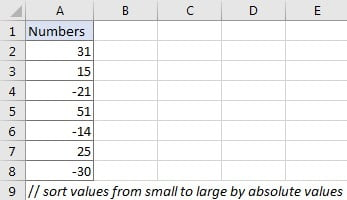We want to sort numbers in “Numbers” column from smallest to largest by absolute values.

## ANALYSIS

If we directly click “Sort Smallest to Largest” button, numbers are sorted by actual values, negative numbers are always the smallest ones. So, if we want to sort them by absolute values, we need to do below two steps:

a. Convert negative numbers to positive number.

b. Sort all positive numbers.

We can apply ABS function to help us solve this problem.

## STEPS

First of all, create a helper column. A helper column can help us save temporary data or simplifies some complex formulas. In this instance, we create a helper column to save absolute values of the given numbers.

a. In this instance, just create the helper column adjacent to “Numbers” column.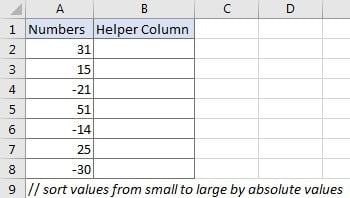b. Enter =ABS(A2) into B3 and press Enter. ABS function is used for returning the absolute value of the given number. The syntax is ABS(number).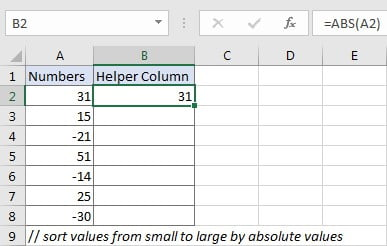c. Drag down the fill handle to copy down this formula from B2 to B8.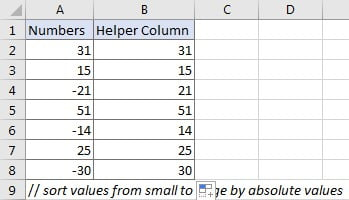d. Select range B2:B8, then click Data > Sort A to Z (Sort Smallest to Largest) in Sort & Filter section.e . Verify that below “Sort Warning” dialog pops up immediately. As we want to sort numbers in “Numbers” column (A column) with the help of helper column (B column), so just keep default option checked, and click “Sort”. If we just want to sort current selection, check on “Continue with the current selection”. The two options are mutually exclusive.f. After clicking “Sort” button on “Sort Warning” dialog, numbers are sorted from smallest to largest properly in both two columns. We can see that in A column negative numbers are still displayed with negative sign.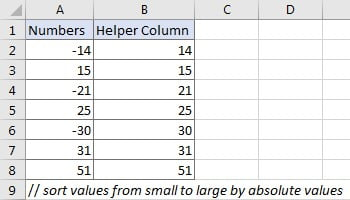g. Delete helper column, now numbers in A column are sorted by absolute values properly as we expect.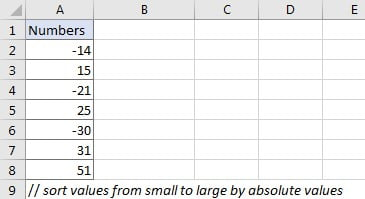### Related Functions

• Excel ABS Function
The Excel ABS function returns the absolute value of a number.The ABS function is a build-in function in Microsoft Excel and it is categorized as a Math and Trigonometry Function.The syntax of the ABS function is as below:=ABS (number)…

Sidebar